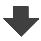Search:

# Reaction of glucose with potassium permanganate and sulfuric acid

The permanganate ion (violet) has the formula $MnO_4^-$. Calculate the oxydation number of manganese !

Let x be the oxidation number of manganese: $x$ $+$ $4(-2)$ $=$ $-1$, so $x$ $=$ $7$

The manganate ion (green) has the formula $MnO_4^{2-}$. Calculate the oxydation number of manganese !

Let x be the oxidation number of manganese: $x$ $+$ $4(-2)$ $=$ $-2$, so $x$ $=$ $6$

The mixed oxide and hydroxide of manganese (brown) has the formula $MnO(OH)_2$. Calculate the oxydation number of manganese!

$x$ $+$ $(-2)$ $+$ $2(-2+1)$ $=$ $0$, so $x$ $=$ $4$

What did happen with manganese during the present reaction?

The manganese has been reduced, it has captured electrons.

Calculate the oxidation number of the colored atoms of glucose and the gluconic acid resulting from this equation!We act as if the most electronegative atom in each bond had alone for himself all the electrons of this bond:So carbon would "have" here 3 electrons in the outermost layer, having 4 he would be neutral (Lewis structure!), so its fictitious charge (oxidation number) is +1So carbon would "have" here 1 electron in the outermost layer, having 4 he would be neutral (Lewis structure!), so its fictitious charge (oxidation number) is +3

What happened to carbon during this reaction?

Carbon was oxidised, so he has lost electrons.